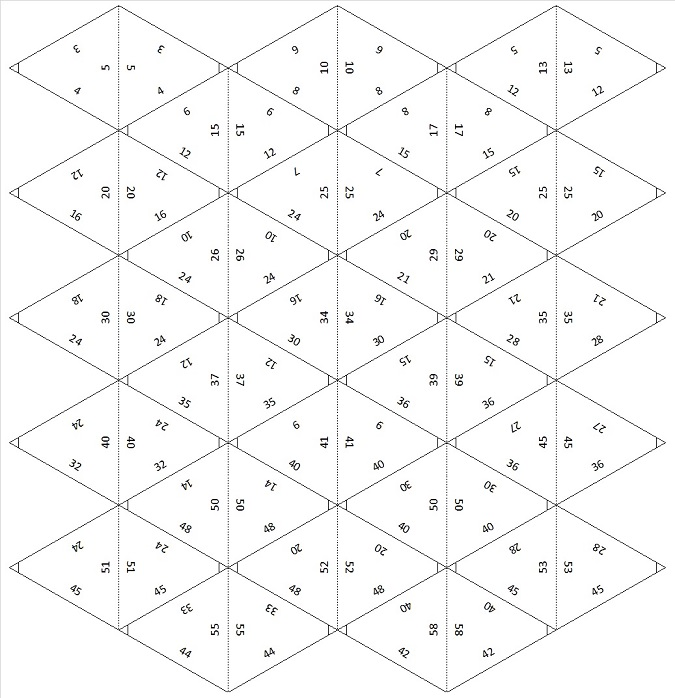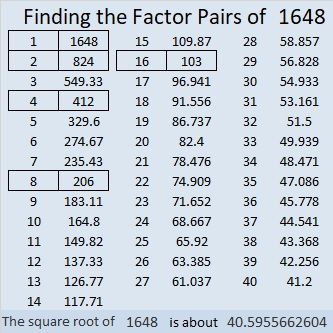# 1648 A Pythagorean Triple Logic Puzzle with a Triangular Card Deck

Contents

### Today’s Puzzle:

It’s been a few years since I’ve made one of these Pythagorean triple logic puzzles. The triangles in it are shaped a little different than in years past because I also wanted to make a deck of Pythagorean triple cards that are shaped like equilateral triangles or at least as close as I can get to equal sides. This puzzle won’t be easy, but do give it a try!

PUZZLE DIRECTIONS: This puzzle is NOT drawn to scale. Although all of the angles may look like 60-degree angles, none of them are. The marked angles are 90 degrees. Lines that look parallel are NOT parallel. Although side lengths look equal, they are NOT equal. Most rules of geometry do not apply here: in fact, non-adjacent triangles in the drawing might actually overlap.

No geometry is needed to solve this puzzle. All that is needed is logic and the table of Pythagorean triples under the puzzle. The puzzle only uses triples in which each leg and each hypotenuse is less than 100 units long. The puzzle has only one solution.Print the puzzles or type the solution in this excel file: 12 Factors 1639-1648

### Triangular Card Deck of Pythagorean Triples:

You can make this deck of 50 playing cards to help you solve the puzzle or perhaps to play a domino-type game. Print each group of 25 cards on a separate sheet of paper. Cut the cards out along the solid lines and fold the cards on the dotted lines. Use a glue stick to keep the front of each card attached to its back. Laminate the cards, if desired. The right angles are the only angles marked on the cards, and the hypotenuses are all along the folded edges. Note: Some sides will not match with any other side in the deck, and the 57-76-95 triangle does not match sides with ANY triangle with a hypotenuse less than 100.### Factors of the Number 1648:

• 1648 is a composite number.
• Prime factorization: 1648 = 2 × 2 × 2 × 2 × 103, which can be written 1648 = 2⁴ × 103.
• 1648 has at least one exponent greater than 1 in its prime factorization so √1648 can be simplified. Taking the factor pair from the factor pair table below with the largest square number factor, we get √1648 = (√16)(√103) = 4√103.
• The exponents in the prime factorization are 4 and 1. Adding one to each exponent and multiplying we get (4 + 1)(1 + 1) = 5 × 2 = 10. Therefore 1648 has exactly 10 factors.
• The factors of 1648 are outlined with their factor pair partners in the graphic below.### More About the Number 1648:

1648 is not the hypotenuse of any Pythagorean triples because none of its prime factors leave a remainder of 1 when divided by 4.

1648 is the difference of two squares three different ways:
413² – 411² = 1648,
208² – 204² = 1648, and
107² – 99² = 1648.

That means 1648 is a leg in THREE Pythagorean triples calculated from
413² – 411², 2(413)(411), 413² + 411²;
208² – 204², 2(208)(204), 208² + 204²; and
107² – 99², 2(107)(99), 107² + 99².

1648 can be expressed as 2(824)(1), 2(412)(2), 2(206)(4), as well as 2(103)(8).

That means 1648 is a leg in FOUR Pythagorean triples calculated from
2(824)(1), 824² – 1², 824² + 1²;
2(412)(2), 412² – 2², 412² + 2²;
2(206)(4), 206² – 4², 206² + 4²; and
2(103)(8), 103² – 8², 103² + 8².

Sometimes those formulas produce duplicate triples, but not this time. 1648 is in SEVEN Pythagorean triples!
1648-339486-339490,
1648-84864-84880,
1648-21186-21250,
1648-678975-678977,
1648-169740-169748,
1648-42420-42452,
1648-10545-10673.

Aren’t you glad that today’s puzzle was limited to triples with hypotenuses less than 100? I certainly wouldn’t want any of those triples with 1648 as a side to be part of any puzzle! But 2-digit sides, 16 and 48, helped to make today’s puzzle, and I do hope you were able to solve it today!

This site uses Akismet to reduce spam. Learn how your comment data is processed.